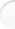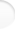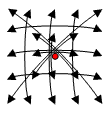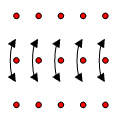Structure of Magic and Semi-Magic Squares, Methods and Tools for Enumeration by Francis Gaspalou1. Enumeration

An enumeration of magic squares of order 5 is possible with existing personal computers, if you use a compiled language and a reduced program.

In September 1997, I drove such an enumeration in 6 hours and half with a reduced program (group G of order 32 and permutation "complement to 26") on a K6 of AMD, running at 200 MHz, under Turbo Pascal. I found the well known total of 2 202 441 792 squares.

In fact, I found 68 826 306 elementary squares by a reduced program/32, and the permutation "complement to 26" allowed to limit enumeration to C3 running from 1 to 13 only (finally I got 36 798 121 elementary squares). For C3=13, it should be theoretically possible to reduce still more the number of elementary squares with the permutation "complement to 26", but the program should be more complicated (we have to consider unchanged elementary squares by this permutation, as symmetrical squares). It should be also useless to evaluate the total number of magic squares (it should be useful only to have a set of elementary squares).
The program has 14 parameters, with 14 imbricate loops, all on the same principle.

I remember that Richard SCHROEPPEL was the first to compute the number of magic squares of order 5 in 1973 (cf. article of Martin GARDNER in Scientific American, January 1976, Volume 234).

 2. The Most Famous Sets Top of the pageSetNumber of
squares
Number of
parameters
Order of
group G
Number of
elementary
squares
Total number
of isomorphic sets
Particular type1 393 92082 0346052 (1)
Symmetrical squares388 35281283 0343 (2)
Pandiagonal squares28 800828 80012 (3)
de la LOUBERE's squares5 76075 76018 (4)
BACHET's squares1 15261 15214 (5)

(1) there is also the set*A
(2) there are also the set*K and the set*K*IT
(3) there is also the set*IT (or the set*A)
(4) see hereafter § 2.4 in fine
(5) see hereafter § 2.5 in fine

For A and IT, I take the same notations as for the 32 transformations of group G for magic squares of order 4 (see Appendix 3, § 1 and Appendix 3, § 2):

 A1 A4 A3 A2 A5 D1 D4 D3 D2 D5 C1 C4 C3 C2 C5 B1 B4 B3 B2 B5 E1 E4 E3 E2 E5

IT
 B2 B1 B3 B5 B4 A2 A1 A3 A5 A4 C2 C1 C3 C5 C4 E2 E1 E3 E5 E4 D2 D1 D3 D5 D4

A

For K, I take the equivalent definition of a transformation on semi-pandiagonal squares of order 4:

 A1 A5 A3 A4 A2 E1 E5 E3 E4 E2 C1 C5 C3 C4 C2 D1 D5 D3 D4 D2 B1 B5 B3 B4 B2

K

For the number of isomorphic sets, the given numbers are minimal numbers, and maybe somebody will find other isomorphic sets.

2.1 Particular type

It is the type:E.g.
 1 17 22 23 2 7 8 20 11 19 12 16 13 10 14 21 15 6 18 5 24 9 4 3 25

It is also a "bordered square" or a "concentric square" (centre is magic), but a bordered square with a fixed type.

2.2 Symmetrical squares

The group G of 128 is made with the classical group of 32 (for all the squares of order 5) and with the two transformations:and2.3 Pandiagonal squares

They can be defined by any intermediate square (I take the first square of BENSON & JACOBY on page 126; the second square is not necessary with my conventions):

 A+a B+b C+c D+d E+e C+d D+e E+a A+b B+c E+b A+c B+d C+e D+a B+e C+a D+b E+c A+d D+c E+d A+e B+a C+b

You have no magic conditions. Therefore, you have 5! =120 solutions for capital letters, 5! solutions too for lower-case letters, k=2 basis; therefore you have a set of 2 * 120 * 120 = 28 800 squares (all the pandiagonal squares are generated with this intermediate square).
It is a set with 4 parameters for capital letters (the 5th follows from them) and 4 parameters for lower-case letters (id°), therefore it is a set with 8 parameters.

You can also define pandiagonal squares by 4 conditions on parallels to the first diagonal which are magic, and 4 conditions on parallels to the second diagonal. You find that gives 6 independent conditions on the 14 classical parameters (of a magic square of order 5) and then you have 8 parameters. For example, with the 8 parameters M=C3, A1=A, E5=Y, B2=G, A5=E, B4=I, A2=B, A4=D, you can calculate all the cells; you find:

 A B 65-A-B-D-E D E -B+M+Y G A+B+D+E-G-I-M I 65-A-D-E-Y B+D+E-G-M 65-A-B-D-E+I-Y M -B-D+G+M+Y A+B+D-I-M -D+G+M A-I+Y B+D-M 65-A-G-M-Y -B+I+M 65-A-E-M-Y D+E-G -B-D+G+I+M A+B-I Y

On the 28 800 pandiagonal squares, the group G is not only of 800 as BENSON & JACOBY thought considering only transpositions. In fact, you can verify that the transformations between the 36 essentially squares given by these authors are good for all the squares. Then the group G is of order 28 800.

2.4 de la LOUBERE's squares

E.g.
 17 24 1 8 15 23 5 7 14 16 4 6 13 20 22 10 12 19 21 3 11 18 25 2 9

I define de la LOUBERE's squares by the intermediate square (coming from this particular square with 1=A+a, 2=A+b,... , but it should be possible to take any other square):

 D+b E+d A+a B+c C+e E+c A+e B+b C+d D+a A+d B+a C+c D+e E+b B+e C+b D+d E+a A+c C+a D+c E+e A+b B+d

The only magic condition is on the second diagonal: 5C = Σ, or C = Σ/5.
You have 4! = 24 solutions for capital letters, 5! = 120 solutions for lower-case letters, k=2 basis; therefore you have a set of 2 * 24 * 120 = 5 760 squares.
Note: W.W. ROUSE BALL & H.S.M. COXETER, in their book Mathematical recreations and essays (University of Toronto Press, 12th edition, 1974, p. 202), speak about 2 880 squares, though only 720 of them are distinct. For me, it is not clear and I think there is a little mistake (there are in fact 5 760 squares or 720 if you consider as a same square the 8 classical variations).
This set is a set with 3 parameters for capital letters and 4 parameters for lower-case letters, therefore with 7 parameters.

You can also define de la LOUBERE's squares by magic relations of definition. For that, you examine what become, in the intermediate de la LOUBERE's square, the 20 lines (5 rows, 5 columns, 5 parallels to the first diagonal and 5 parallels to the second diagonal) of the above pandiagonal intermediate square. You find 10 supplementary conditions:
• 5 parallels to the first diagonal are magic,
• 5 other conditions (which are the parallels to the first diagonal of the above pandiagonal square):
A3+C4+E5+B1+D2=S
E3+C2+A1+D5+B4=S
A4+D3+B2+E1+C5=S
B5+E4+C3+A2+D1=S
C1+A5+D4+B3+E2=S.
Among these 10 supplementary conditions, you find there are only 7 independent conditions. Therefore, the set of de la LOUBERE's squares is a set with 14 - 7 = 7 parameters.
With the 7 parameters C3=M, A1=A, E5=Y, B2=G, A5=E, E1=U, B4=I, you can calculate all the cells; you find:

 A 65-A-G+I-M-U-Y -E+G+U -I+M+Y E 65-A-G-U-Y G 65-E-2*I-M-U+Y I A-E+I+M+2*U-65 -E+G+I -I+U+Y M A+2*E+I+M+U-65 130-A-E-G-I-2*M-2*U-Y E-I+Y 65-E-I-M-U A+E+2*I+M+U-65 65-A-G-M-Y -E+G+M U A+E+I+2*M+U-65 65-A+E-G-M-U-Y 65-2*E+G-I-M-U Y

There are 8 isomorphic sets. As a matter of fact, among the 32 transformations of the classical group of 32, the 4 transformations {I, R2, IT*A, EX*A} belongs to group G of de la LOUBERE's squares, therefore you have the 32/4 = 8 isomorphic sets:
set, set*V, set*G, set*R3, set*IT, set*X, set*M, set*T.

Note that the 5 760 de la LOUBERE's squares are isomorphic to a part of the 28 800 pandiagonal squares: the pandiagonal squares with condition A3+B1+C4+D2+E5=65 (or A3+B5+C2+D4+E1=65). The first condition comes from the position of letter C in the pandiagonal intermediate square above (and the second condition from the position of letter c).

2.5 BACHET's squares

E.g.
 23 6 19 2 15 10 18 1 14 22 17 5 13 21 9 4 12 25 8 16 11 24 7 20 3

I define BACHET's squares by the intermediate square coming from this particular square (but it should be possible to take any other square):

 E+c B+a D+d A+b C+e B+e D+c A+a C+d E+b D+b A+e C+c E+a B+d A+d C+b E+e B+c D+a C+a E+d B+b D+e A+c

The magic conditions are: 5C = Σ in the second diagonal and 5c = σ in the first diagonal.
You have 4! = 24 solutions for capital letters, 4! = 24 solutions for lower-case letters, k=2 basis; therefore you have a set of 2 * 24 * 24 = 1 152 squares.
This set is a set with 3 parameters for capital letters and 3 parameters for lower-case letters, therefore with 6 parameters.

You can also define BACHET's squares by the 10 supplementary conditions (here also, you examine what become the 20 lines of the above pandiagonal intermediate square):
• 5 conditions of de la LOUBERE's squares:
A3+C4+E5+B1+D2=S
E3+C2+A1+D5+B4=S
A4+D3+B2+E1+C5=S
B5+E4+C3+A2+D1=S
C1+A5+D4+B3+E2=S
• 5 new relations:
A1+B3+C5+D2+E4=S
A2+B4+C1+D3+E5=S
A3+B5+C2+D4+E1=S
A4+B1+C3+D5+E2=S
A5+B2+C4+D1+E3=S
Among these 10 supplementary conditions, you find there are only 8 independent conditions. Therefore, the set of BACHET's squares is a set with 14 - 8 = 6 parameters.
With the 6 parameters A1=A, E5=Y, B2=G, A5=E, E1=U, B4=I, you can calculate all the cells; you find:

 A 39-A-G+U-Y G+I-13 39-E-I-U+Y E 39-A+E-G-Y G U+Y-13 I 39+A-E-I-U 39-E+G-I-U E+Y-13 13 A+U-13 39-A-G+I-Y I+Y-13 52-E-I-U A+E-13 52-A-G-Y G+U-13 U A+I-13 91-A-E-G-I-U-Y E+G-13 Y

There are 4 isomorphic sets. As a matter of fact, among the 32 transformations of the classical group of 32, all the 8 transformations of the octic group belongs to group G of BACHET's squares, therefore you have the 32/8 = 4 isomorphic sets:
set, set*IT, set*A, set*IT*A

The 1 152 BACHET's squares are isomorphic to a part of the 28 800 pandiagonal squares: the pandiagonal squares with the 2 conditions A3+B1+C4+D2+E5=65 and A3+B5+C2+D4+E1=65 (cf. position of letters C and c in the pandiagonal intermediate square above). The 1 152 BACHET's squares are also isomorphic to a part of the 5 760 de la LOUBERE's squares.

Note: I found that the following de la HIRE's square

 18 4 21 10 12 2 23 9 11 20 25 7 13 19 1 6 15 17 3 24 14 16 5 22 8

belongs to BACHET's set ( with A=5, B=0, C=10, D=20, E=15; a=4, b=5, c=3, d=1, e=2).
This de la HIRE's square comes from propositions X and XIV of this author (Reference: W. ROUSE BALL, Récréations mathématiques et problèmes des temps anciens et modernes, Librairie scientifique A . Hermann, 1926, p. 163).

It should be interesting to see if it is true for all the de la HIRE's squares, and more generally it should be interesting to calculate sets of squares given by other methods as POIGNARD's, MARGOSSIAN's, ARNOUX's,...

 3. Other Sets Top of the pageThere are many other sets that can be computed.

• To distribute the magic squares of order 5 in different "types" is practically impossible. But you can do this for some particular sets. For example, I found 225 different types of pandiagonal squares and I computed the number of squares (not only pandiagonal) in each elementary type.

• Another set of squares is very important by its population: it is the set of what I call the "quasi-symmetrical" squares, that is to say squares with sum of opposite cells being constant except for some ones, but compulsorily for cells on the two diagonals, the middle row and the middle column. Naturally, centre has to be odd. Example:

 1 25 19 13 7 14 8 2 21 20 22 16 15 9 3 10 4 23 17 11 18 12 6 5 24

where all the sums of opposite cells are equal to 25 except for A2+E4 and B5+D1.

It is a set with 11 parameters and you can study different families.

• I searched the equivalent for order 5 of the 3 456 semi-pandiagonal set for order 4. More exactly, I searched the orthogonal squares to Latin diagonal squares (see § 4 hereafter).

Note: you can take another definition of semi-pandiagonal squares. You can search the space of definition of transformation K (cf. § 2 above), i.e. the set A2+B1+C3+D5+E4=65. It is a set with 13 parameters. If I have time, I will do this enumeration to compare result to orthogonal squares to Latin diagonal squares. Maybe it is necessary to add other conditions coming from other specific transformations as K.
If you have the 2 conditions A2+B1+C3+D5+E4=65 and A4+B5+C3+D1+E2=65, then C3=13 (A2+A4+B1+B5+D1+D5+E2+E4=65+3*C3 for all the magic squares of order 5).
It should be interesting also to search "quasi symmetrical" squares with condition A2+B1+C3+D5+E4=65 (set with 10 parameters).

• In the same order of idea, I think to calculate all the semi-regular squares should be interesting. Among these squares, we know already the pandiagonal set (regular squares), the de la LOUBERE's set, the BACHET's set, and all the isomorphic sets.

I calculated a part of these semi-regular squares: the set of the orthogonal squares to Latin magic squares: I found 29 elementary squares and a total of 1 198 720 squares (among these squares, 410 880 are orthogonal squares to Latin diagonal squares: see § 4 hereafter).

• Note: I draw the attention to the Bernard GERVAIS's book, Les carrés magiques de 5, Eyrolles 1997. This author has studied "magic mosaics" in giving a colour to cells with odd numbers and another colour to cells with even numbers. He found 721 different mosaics (and different families among these mosaics).

 4. Orthogonal Squares to Latin Diagonal Squares Top of the pageI remember that a Latin diagonal square is a square with two Latin diagonals. A Latin diagonal square is a specific kind of Latin magic square: in a Latin magic square, the two diagonals are magic, but not necessarily Latin.

4.1 Enumeration of Latin diagonal squares

I searched elementary Latin diagonal squares with 1, 2, 3, 4, 5 on the first row. I found 8 elementary solutions (program with 10 parameters). This number has to be multiplied by 5! = 120 to get the total number of solutions: there are 960 Latin diagonal squares of order 5.

If you apply the group G of order 32 and the 120 permutations (1 2 3 4 5), you will see that the 8 elementary solutions can be reduced to 2 (the first 2 among 8 for example):

 1 2 3 4 5 5 3 4 1 2 4 5 2 3 1 2 4 1 5 3 3 1 5 2 4
and
 1 2 3 4 5 5 3 1 2 4 2 5 4 3 1 4 1 2 5 3 3 4 5 1 2

Then, by application of group G of 32 and permutations,
 the first square gives (32 * 120)/8 = 480 induced squares, the second square (32 * 120)/8 = 480 «            « total 960 Latin diagonal squares

(the first square is the same - after permutation - when you apply 8 transformations making a subgroup of group G of 32,
and the second square is the same - still after permutation - when you apply another subgroup of order 8).

4.2 Enumeration of orthogonal squares to Latin diagonal squares

Number of orthogonal squares to these 2 elementary squares: 536 and 320 given by my program with 14 parameters.

Therefore there are:
 (480 * 536) + (480 * 320) = 410 880 orthogonal squares to Latin diagonal squares(or semi-regular squares with a regular lower-case square).

The second basis gives the same squares. See explanation and comparison with other orders in next Appendix 5, § 1.2.

4.3 Some sets among the Total

Among the 410 880 squares, you have:
• 2*28 800 pandiagonal squares with their isomorphic set*IT
• 8*5 760 de la LOUBERE's squares with their isomorphic sets
that is to say 103 680 squares already.

The analysis of the remaining squares should be interesting, in particular identification of new sets.
For example, there are symmetrical squares which are not pandiagonal nor de la LOUBERE's (with isomorphic sets).
From a given square, you can also build the intermediate square obtained with the classical basis (1,2,...,5;0,5,...,20) and generate all a set of squares. In that way, you decompose the 410 880 squares in sets of intermediate squares.

I began to do this, but I did not finish and I haven't results to show here yet.